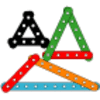#### You may also like### Cubes

How many faces can you see when you arrange these three cubes in different ways?### I'm Eight

Find a great variety of ways of asking questions which make 8.### Let's Investigate Triangles

Vincent and Tara are making triangles with the class construction set. They have a pile of strips of different lengths. How many different triangles can they make?

# That Number Square!

##### Age 5 to 11 Challenge Level:
We had a lot of ideas coming from Holy Trinity Primary School in Halstead, Essex.

Firstly, Kellum, Harry and Aaron wrote:

First we filled in the tens column [down] because we think it is the quickest method and the easiest.
After we sorted the tens column out, we went to the units column.  The units column was found the option for us to use easily because it was only a one-digit number.
Next we went down to the 9s column and so on we finished the 9s column. Then 8s, 7s, 6s, 5s,
4s, 3s, 2s and 1s columns.

Example
1  2  3  4  5  6  7   8   9  10
12 13 14 15 16 17 18 19 20

This is what Brandon, Harley and Scott said:

First, we looked at the numbers we had to work with. We had numbers 0 - 99. After that, we sorted them into groups.
Group one consisted of the outline numbers, and group two consisted of the middle numbers. Outline numbers are the numbers at the top, sides and bottom. On the left hand side, the numbers go down in 10s, starting from 0; on the right hand side the numbers go down in 10s, starting from 9. For example, 9, 19, 29 and so on. On the top are consecutive numbers; 0 - 9, left to right. Finally, on the bottom, are consecutive numbers, 90 - 99, left to right.
We placed all of the outline numbers on the outside of the grid so then we had an idea where the middle numbers needed to be placed. We placed the middle numbers by working from the outside numbers; hence why we did the outside numbers first. For example, take the number 60. Then you can work your way into the middle by counting to your right.

Jack, Eddy, Eddie and Lily wrote:

First we picked up all the number squares and found all the corner numbers (0, 9, 90 and 99).
We filled in all the numbers between 0 and 9, then 9 to 99, and lastly we did the two corners 0 to 90 and 90 to 99. Then we start.
Look at 1 on the grid, next look down and add 10 onto the number every time until we got up to the bottom of the grid.
Next you look to the next column and do exactly the same.
Carry on doing this method until you get up to 99.
This is a good method because it’s a quick and easy way to complete the task.

Next Paris, Billy and Megan sent in their ideas:

1] First one person starts putting the numbers in piles.
2] The piles that we put them into are 10, 20, 30 and so on.
3] The second person was putting the piles into columns of the right order.
4] The third person was sticking the columns back onto the wall in the right order.

Finally Finley and Ruby wrote:

First we turned all of the numbers over and spread them out so we could see the numbers.
Next we put the numbers 0-9 across the top.
After that we filled the perimeter then we put all the numbers that end in five down the middle in a straight line.
Finally, we found all the numbers that ended in a zero which we put down the side.

Butterfly Class at Bredgar Cof E Primary School wrote the following:

We used lots of strategies. Here are some of our favourites:
1. Start with the tens column and count in ten (we learnt that in year 1). Then go to the first column and fill in going down by adding 1 more to the last ten’s number.

2. Start along the top row. Fill in 1 to 10, then you can fill in each column by adding 10 more each time.

3. Some of us counted in ones because we can do this super-fast.

Thank you to everyone who sent in their ideas, it's good to see that there is not just one way of doing this as is the case for so much mathematics.